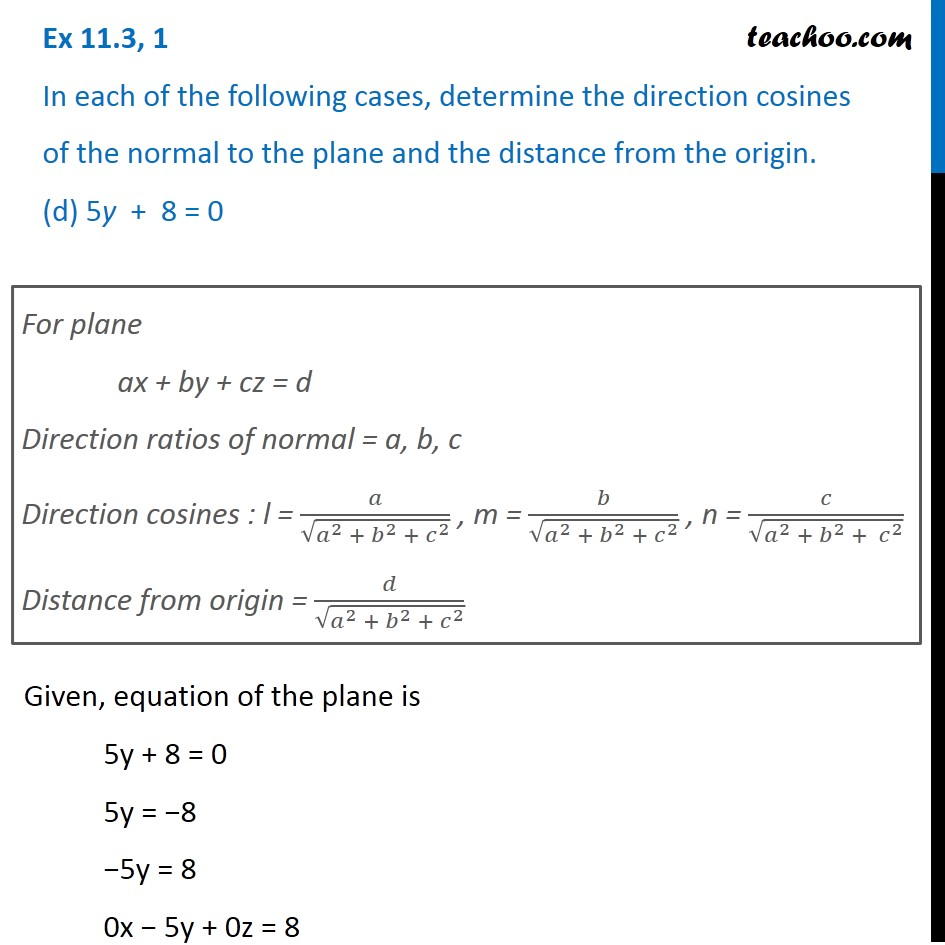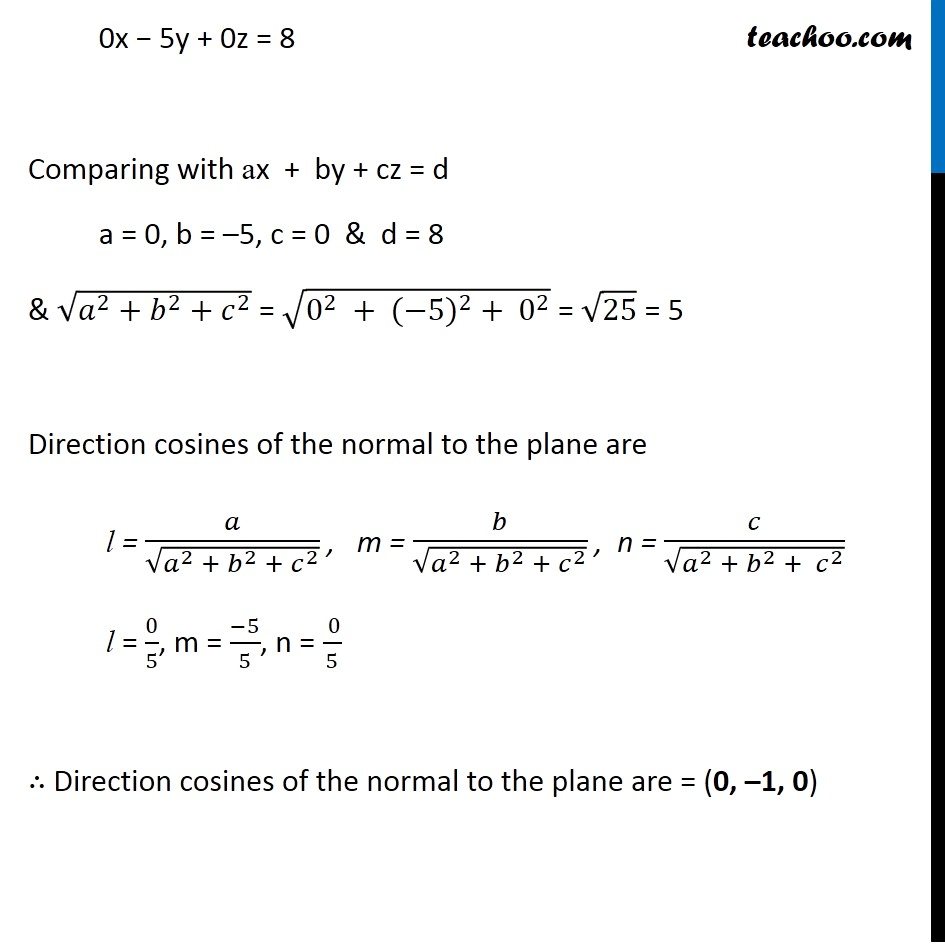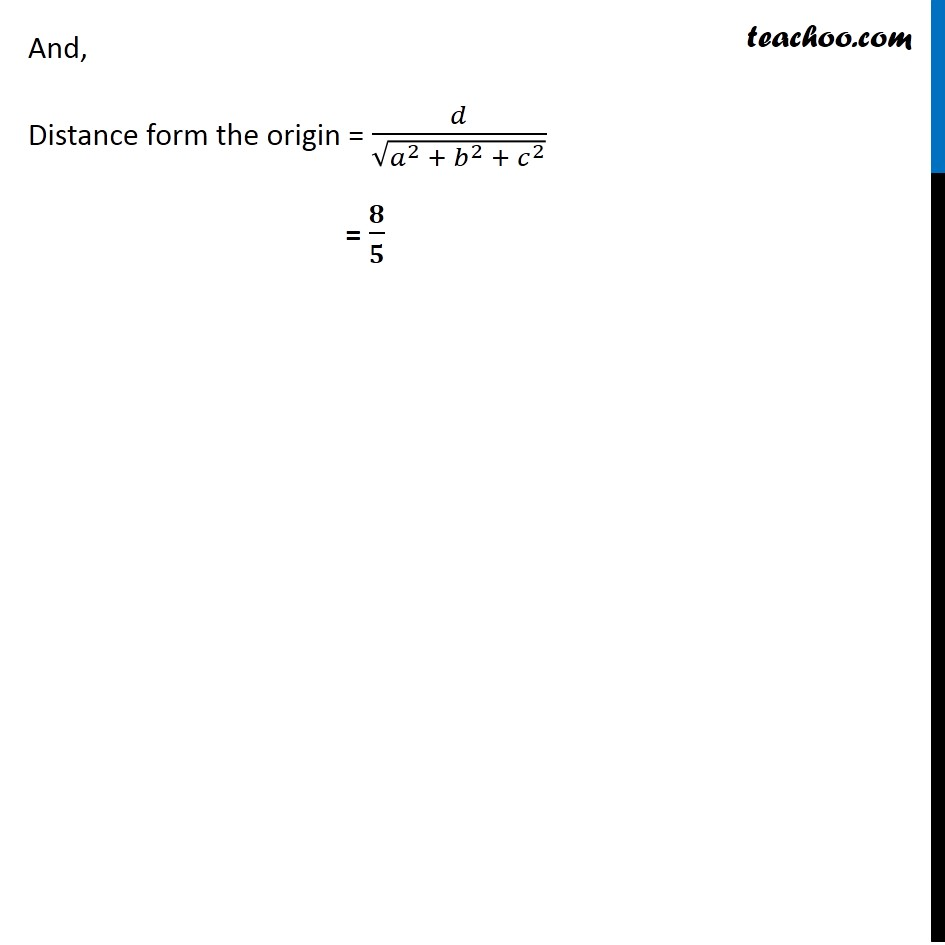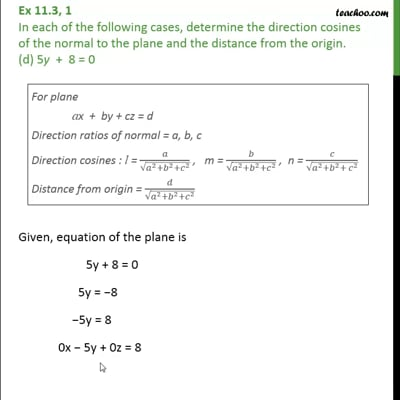Ex 11.3

Chapter 11 Class 12 Three Dimensional Geometry
Serial order wiseThis video is only available for Teachoo black users

Introducing your new favourite teacher - Teachoo Black, at only ₹83 per month

### Transcript

Ex 11.3, 1 In each of the following cases, determine the direction cosines of the normal to the plane and the distance from the origin. (d) 5y + 8 = 0 For plane ax + by + cz = d Direction ratios of normal = a, b, c Direction cosines : l = 𝑎/√(𝑎^2 + 𝑏^2 +〖 𝑐〗^2 ) , m = 𝑏/√(𝑎^2 + 𝑏^2 + 𝑐^2 ) , n = 𝑐/√(𝑎^2 + 𝑏^2 + 𝑐^2 ) Distance from origin = 𝑑/√(𝑎^2 + 𝑏^2 + 𝑐^2 ) Given, equation of the plane is 5y + 8 = 0 5y = −8 −5y = 8 0x − 5y + 0z = 8 0x − 5y + 0z = 8 Comparing with ax + by + cz = d a = 0, b = –5, c = 0 & d = 8 & √(𝑎^2+𝑏^2+𝑐^2 ) = √(0^2 + 〖(−5)〗^2 + 0^2 ) = √25 = 5 Direction cosines of the normal to the plane are l = 𝑎/√(𝑎^2 + 𝑏^2 + 𝑐^2 ) , m = 𝑏/√(𝑎^2 + 𝑏^2 + 𝑐^2 ) , n = 𝑐/√(𝑎^2 + 𝑏^2 + 𝑐^2 ) l = 0/5, m = (−5)/5, n = ( 0)/5 ∴ Direction cosines of the normal to the plane are = (0, –1, 0) And, Distance form the origin = 𝑑/√(𝑎^2 + 𝑏^2 + 𝑐^2 ) = 𝟖/𝟓